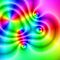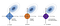# An Introduction to Complex Analysis and Applications

## How imaginary numbers have a real impact.Figure 1: A representation of a Complex Function. These can be created once you understand what a complex function is. The Color represents the argument of the complex function, while the brightness/hue is represented by the magnitude. Image Citation: Creative Commons.

# Introduction:

• We maximise parameters given observed data, that is, we want to find what the parameters should be to result in generating the data we observe with the highest probability. …Figure 1: An image visualising how ordinary regression compares to the Lasso, the Ridge and the Elastic Net Regressors. Image Citation: Zou, H., & Hastie, T. (2005). Regularization and variable selection via the elastic net.

# A Quick Tour of some Alternate Programming Languages used in Data Science and Machine Learning.

## And why you should consider learning one.